CBSE Class 12 Sample Paper for 2022 Boards (MCQ Based - for Term 1)

Class 12
Solutions of Sample Papers and Past Year Papers - for Class 12 Boards

## (c) infinitely many points  (d) two points only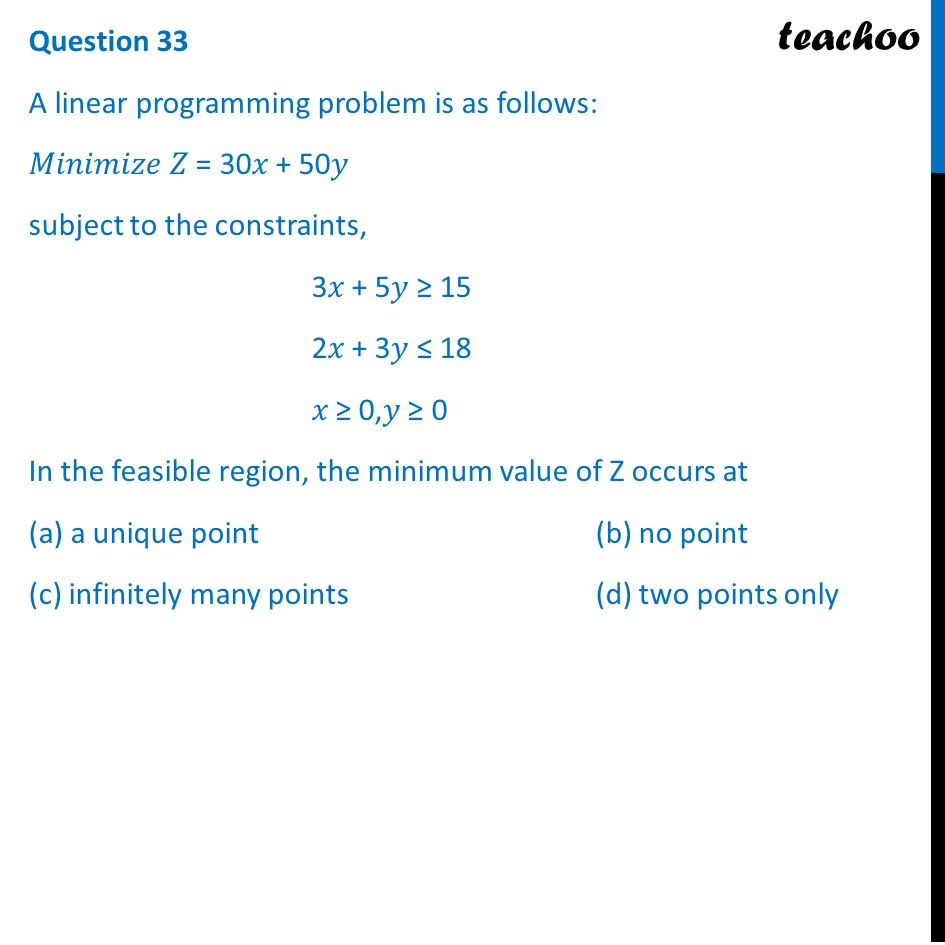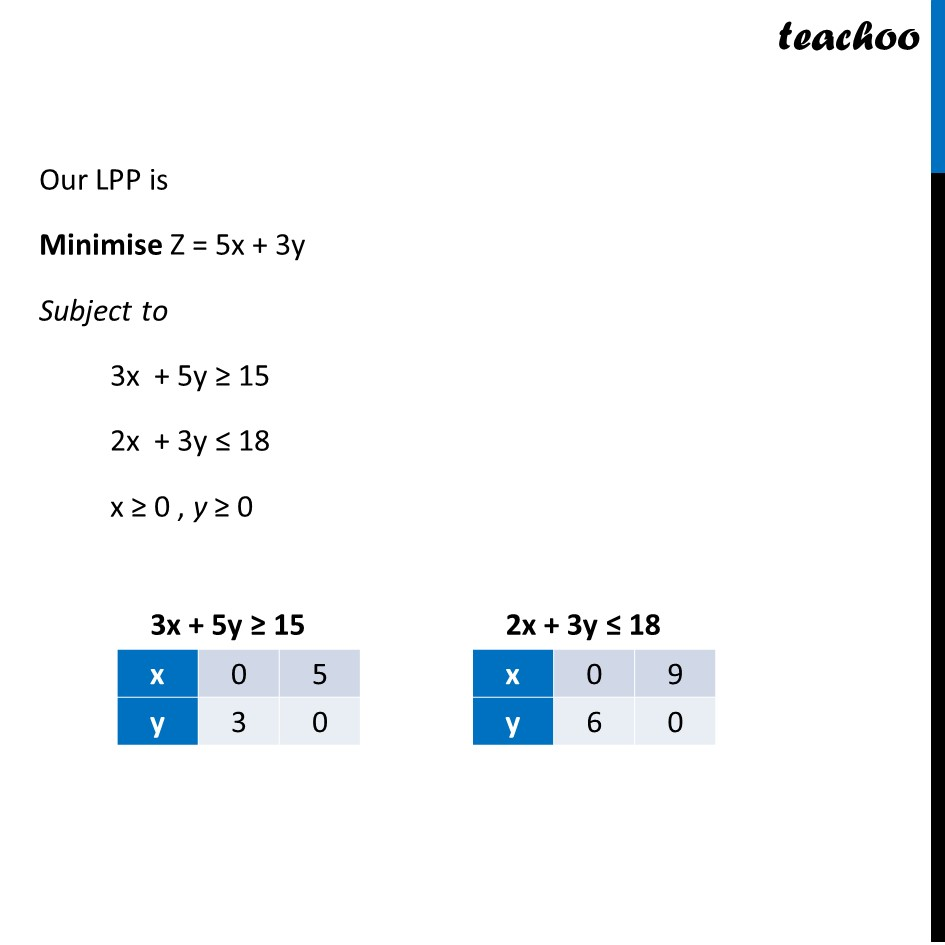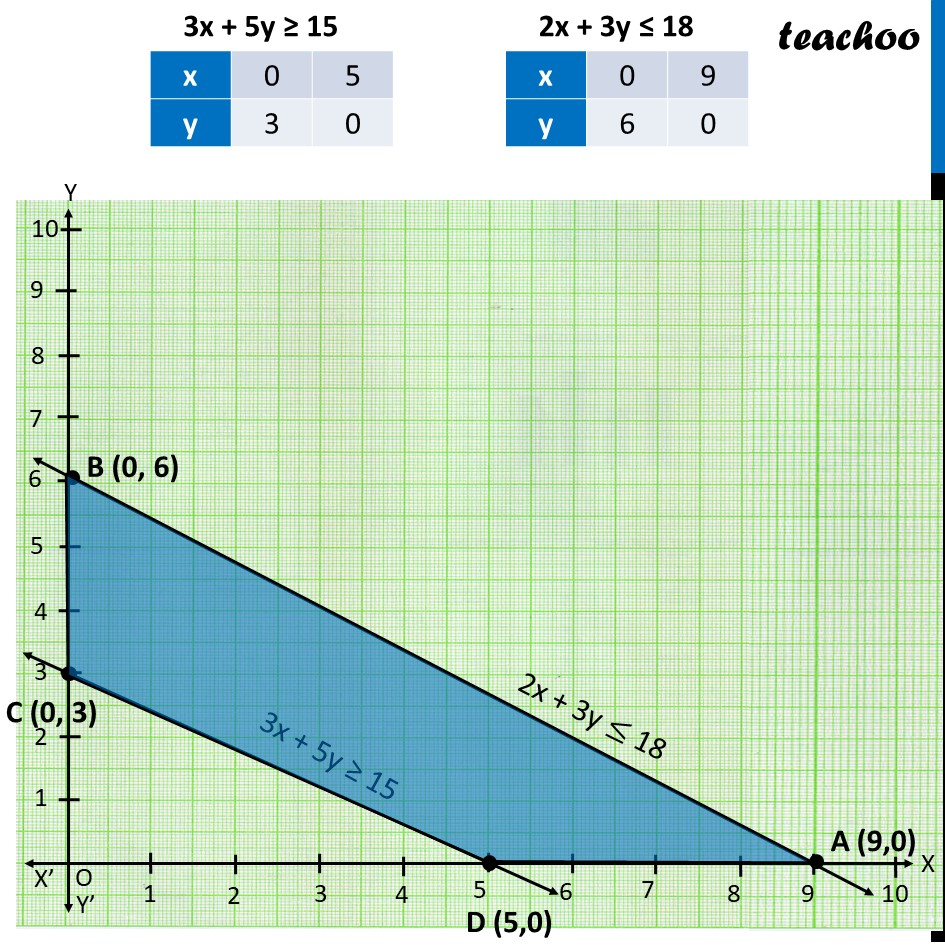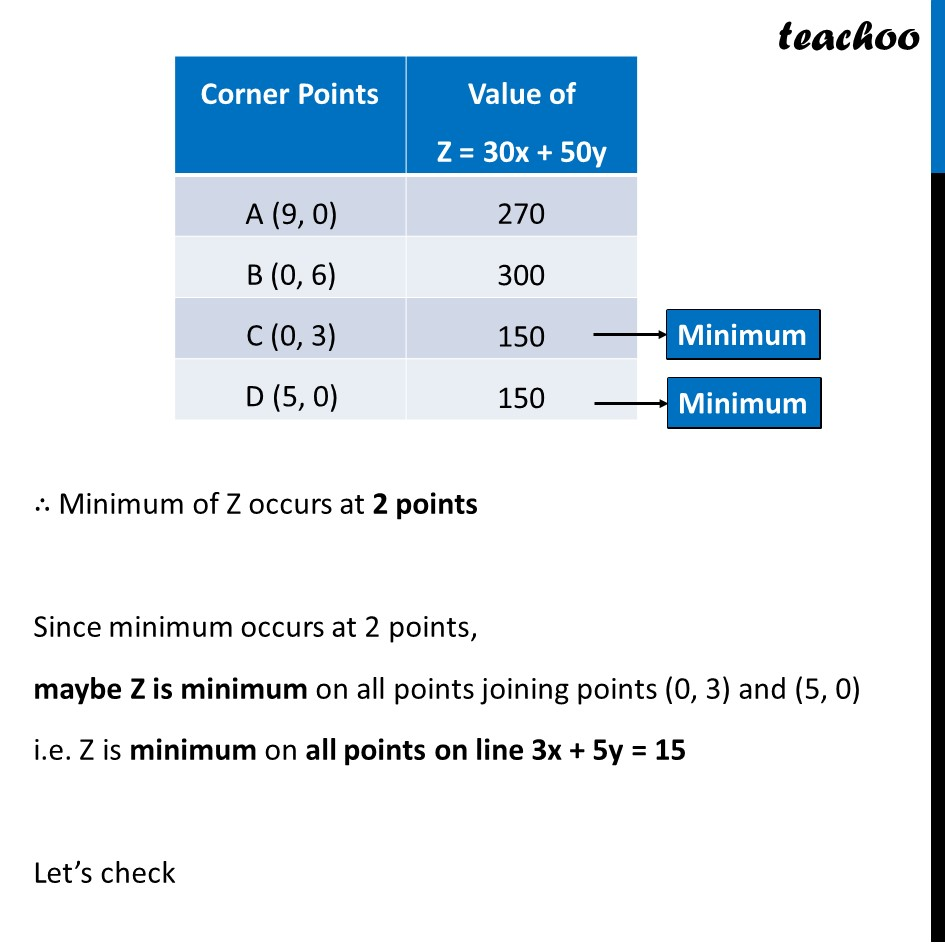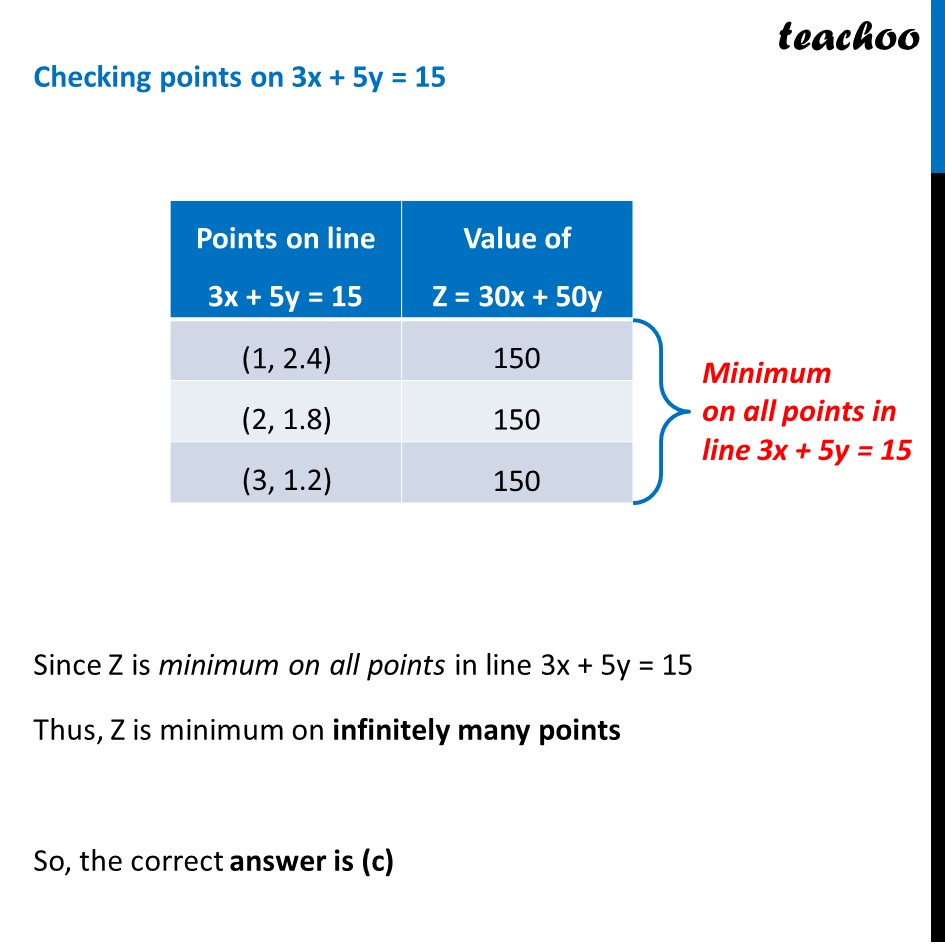### Transcript

Question 33 A linear programming problem is as follows: 𝑀𝑖𝑛𝑖𝑚𝑖𝑧𝑒 𝑍 = 30𝑥 + 50𝑦 subject to the constraints, 3𝑥 + 5𝑦 ≥ 15 2𝑥 + 3𝑦 ≤ 18 𝑥 ≥ 0,𝑦 ≥ 0 In the feasible region, the minimum value of Z occurs at (a) a unique point (b) no point (c) infinitely many points (d) two points only Our LPP is Minimise Z = 5x + 3y Subject to 3x + 5y ≥ 15 2x + 3y ≤ 18 x ≥ 0 , y ≥ 0 ∴ Minimum of Z occurs at 2 points So, the correct answer is (d)